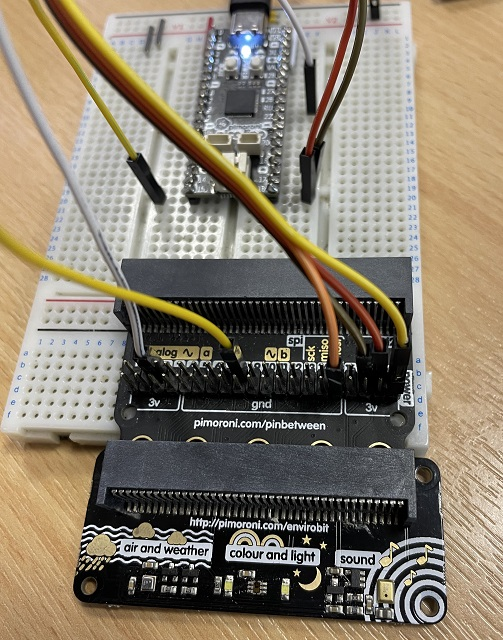# Raspberry Pi Pico Pimoroni enviro:bit

The enviro:bit is a compact but powerful sensor board from Pimoroni. It has a microphone for detecting sound, a TCS3472 light and colour sensor and a BME280 temperature, pressure and humidity sensor.You need to connect the 3V and GND pins to power pins on the Pico. You also need to connect the I2C pins (19 SCL, 20 SDA) from the micro:bit to two of the many I2C pins of the Pico. I connected 19 to GP17 and 20 to GP16 for my test circuit. You also need to connect pin0 from the micro:bit to an ADC pin on the Pico. I used GP26 for this. There is an LED for the colour sensor. This is on pin8 of the micro:bit and I connected it to GP15.

My approach to this was to copy the Pimoroni libraries and make the minor adjustments needed for them to work on the Pico. They were written to be as compact as possible given the memory limitations of the micro:bit.

## Sound

In this program, I am testing out the single and double clap code from the Pimoroni library. I played around with the numbers from the original library to get this working reasonably.

```from machine import ADC
from time import sleep, ticks_ms, ticks_diff
from micropython import const

_offset = const(36000)

def wait_for_clap(timeout = 1000, sensitivity = 15000):
start = ticks_ms()
while ticks_diff(ticks_ms(), start) < timeout:
return True
return False

def wait_for_double_clap(timeout = 1000, spread = 500, sensitivity = 15000):
clap_once = None
start = ticks_ms()
while ticks_diff(ticks_ms(), start) < timeout:
pass
sleep(0.1)
if clap_once is not None and ticks_diff(ticks_ms(), clap_once) < spread:
return True
else:
clap_once = ticks_diff
return False

# single clap
print("Clap")

while True:
if wait_for_clap():
print("Clapped")
break

sleep(3)
# double clap
print("Clap Twice")
while True:
if wait_for_double_clap():
print("Double clapped")
break
```

In this next program, I turned the LED on and off using double claps.

```from machine import ADC, Pin
from time import sleep, ticks_ms, ticks_diff
from micropython import const

_offset = const(36000)

led = Pin(15, Pin.OUT)

def wait_for_clap(timeout = 1000, sensitivity = 15000):
start = ticks_ms()
while ticks_diff(ticks_ms(), start) < timeout:
return True
return False

def wait_for_double_clap(timeout = 1000, spread = 500, sensitivity = 15000):
clap_once = None
start = ticks_ms()
while ticks_diff(ticks_ms(), start) < timeout:
pass
sleep(0.1)
if clap_once is not None and ticks_diff(ticks_ms(), clap_once) < spread:
return True
else:
clap_once = ticks_diff
return False

while True:
if wait_for_double_clap():
print("Double clapped")
led.toggle()
```

## Colour And Light

Here is the converted library. I saved this as tcs3472.py.

```from micropython import const
import struct

_level = 65.535

class TCS3472:
def __init__(self, i2c, led):
self.i2c = i2c
self.led = led

def scaled(self):
crgb = self.raw()
if crgb > 0:
return tuple(float(x) / crgb for x in crgb[1:])

return (0,0,0)

def rgb(self):
return tuple(int(x * 255) for x in self.scaled())

def light(self):
return self.raw()

def brightness(self, level=_level):
return int((self.light() / level))

def valid(self):

def raw(self):

def set_leds(self, state):
self.led.value(state)
```

And this was some code that can be used for testing the colours.

```from machine import Pin, I2C
from time import sleep
from tcs3472 import TCS3472

i2c = I2C(0,sda=Pin(16), scl=Pin(17))
led = Pin(15, Pin.OUT)

t = TCS3472(i2c, led)

while True:
t.set_leds(1)
sleep(0.2)
r, g, b = t.rgb()
t.set_leds(0)
print("#{:02x}{:02x}{:02x}".format(r, g, b))
sleep(0.5)
```

## Temperature, Pressure and Altitude

Here is the library, with minor adjustments.Save to the libraries folder as bme280.py.

```from micropython import const
from time import sleep
import struct
import gc

# 500ms standby time, 16 filter coef
CONFIG = const(0b10010000)

# x16 oversampling, normal mode
CTRL_MEAS = const(0b10110111)

# x16 humidity oversampling
CTRL_HUM = const(0b00000101)

R_CHIPID = const(0xD0)
R_VERSION = const(0xD1)
R_SOFTRESET = const(0xE0)
R_CONTROL = const(0xF4)
R_HCONTROL = const(0xF2)
R_CONFIG  = const(0xF5)
R_STATUS = const(0xF3)

class BME280():
def __init__(self, i2c):
self.i2c = i2c
self._temperature = 0
self._pressure = 0
self._altitude = 0
self._qnh = 1013.25 # hPa

self._w(R_SOFTRESET, 0xb6)
sleep(0.2)
self._w(R_HCONTROL, CTRL_HUM)
sleep(0.2)
self._w(R_CONTROL, CTRL_MEAS)
sleep(0.2)
self._w(R_CONFIG, CONFIG)
sleep(0.2)

def set_qnh(self, qnh):
self._qnh = qnh

def temperature(self):
self.update()
gc.collect()
return self._temperature

def pressure(self):
self.update()
gc.collect()
return self._pressure

def humidity(self):
self.update()
gc.collect()
return self._humidity

def altitude(self):
self.update()
gc.collect()
return self._altitude

self.update()
gc.collect()
return self._temperature, self._pressure, self._humidity, self._altitude

def _w(self, reg, value):

def update(self):
dig_T1, dig_T2, dig_T3, \
dig_P1, dig_P2, dig_P3, \
dig_P4, dig_P5, dig_P6, \
dig_P7, dig_P8, dig_P9, \
_, \
dig_H1, dig_H2, dig_H3, \
reg_E4, reg_E5, reg_E6, \
dig_H6 = struct.unpack("<HhhHhhhhhhhhbBhBbBbb", self.compensation)

dig_H4 = (reg_E5 & 0x0f) | (reg_E4 << 4)
dig_H5 = (reg_E5 >> 4) | (reg_E6 << 4)

if dig_H4 & (1 << 12):
dig_H4 -= 1 << 12
if dig_H5 & (1 << 11):
dig_H5 -= 1 << 12

raw_temp=(raw<<12)|(raw<<4)|(raw>>4)
raw_press=(raw<<12)|(raw<<4)|(raw>>4)
raw_hum=(raw<<8)|raw

var1=(raw_temp/16384.0-dig_T1/1024.0)*dig_T2
var2=(raw_temp/131072.0-dig_T1/8192.0)*(raw_temp/131072.0-dig_T1/8192.0)*dig_T3
temp=(var1+var2)/5120.0
t_fine=(var1+var2)

var1=t_fine/2.0-64000.0
var2=var1*var1*dig_P6/32768.0
var2=var2+var1*dig_P5*2
var2=var2/4.0+dig_P4*65536.0
var1=(dig_P3*var1*var1/524288.0+dig_P2*var1)/524288.0
var1=(1.0+var1/32768.0)*dig_P1
press=1048576.0-raw_press
press=(press-var2/4096.0)*6250.0/var1
var1=dig_P9*press*press/2147483648.0
var2=press*dig_P8/32768.0
press=press+(var1+var2+dig_P7)/16.0

var1 = t_fine - 76800.0
var2 = dig_H4 * 64.0 + (dig_H5 / 16384.0) * var1
var3 = raw_hum - var2
var4 = dig_H2 / 65536.0
var5 = 1.0 + (dig_H3 / 67108864.0) * var1
var6 = 1.0 + (dig_H6 / 67108864.0) * var1 * var5
var6 = var3 * var4 * (var5 * var6)

h = var6 * (1.0 - dig_H1 * var6 / 524288.0)
h = max(0, min(100, h))

self._temperature = temp
self._pressure = press / 100.0
self._humidity = h
self._altitude = 44330.0 * (1.0 - pow(self._pressure / self._qnh, (1.0/5.255)))
```

Here is some test code,

```from machine import Pin, I2C
from time import sleep
from bme280 import BME280

i2c = I2C(0,sda=Pin(16), scl=Pin(17))

b = BME280(i2c)

while True:
t, p, h, a = b.read_all()
print("T: {t}, P: {p}, H: {h}, A: {a}".format(t=t,p=p,h=h,a=a))
sleep(1)
```